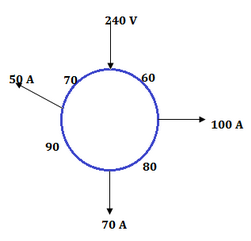# Boosters & Ring distributor of Electrical Power - MCQs with Answers

## Boosters & Ring distributor of Electrical Power - MCQs with Answers

Q1. What are boosters?

a. Is a high voltage and low circuit machine.
b. Is a series wound dc generator driven by dc shunt motor
c. Is a low voltage and high current generator operating on straight or linear portion of its voltage current characteristics
d. Both (b) and (c).

ANSWER: d. Both (b) and (c)

Q2. Why are the boosters inserted in the circuit?

a. Reduce current.
b. Increase current.
c. Reduce voltage drop.
d. Compensate for voltage drop.

ANSWER: d. Compensate for voltage drop.

Q3. Why are the floating neutral in a three phase supply considered undesirable?

a. High voltage across the load.
b. Low voltage across the load.
c. Unequal line voltages across the load.
d. None of above

ANSWER: c. Unequal line voltages across the load.

Q4. A 300m ring distributor has loads as shown in the fig. The distances are in metres. The resistance of each conductor is 0.2 W per 1000 metres and loads are tapped off at B, C, D. If the distributor is fed at A at 240 V, what will be the voltage at B?a. 220.6 V
b. 236.9 V
c. 240.6 V
d. 235.97 V